# 1 Overview:

Item response theory (IRT) is a paradigm for investigating the relationship between an individual’s response to a single test item and their performance on an overall measure of the ability or trait that item was intended to measure. Many models exist in the IRT field for evaulating how well an item captures an underlying latent trait, but some of the most popular IRT models are logistic IRT models. This tutorial will introduce you to 1-parameter, 2-parameter, and 3-parameter logistic IRT models.

For each model you will create:

• Summary plots
• Estimate latent ability scores
• Test the fit of the model

# 2 1-parameter logistic model (1PL)

At the core of all the IRT models presented in this tutorial is the item response function (IRF). The IRF estimates the probability of getting an item “correct” (i.e., $$X=1$$) as a function of item characteristics and the individual’s latent trait/ability level ($$\theta$$). These item response functions are defined by a logistic curve (i.e., an “S”-shape from 0-1).

The 1PL (also called the Rasch model) IRT model describes test items in terms of only one parameter, item difficulty, $$b$$. Item difficulty is simply how hard an item is (how high does the latent trait ability level need to be in order to have a 50% chance of getting the item right?). $$b$$ is estimated for each item of the test.

The item response function for the 1PL model is given below:

$P(X=1|\theta,b)=\frac{e^{(\theta-b)}}{1+e^{(\theta-b)}}$ ##Step 0: Read in the data We will use (simulated) results from N=500 individual taking a 10-item test (V1-V10). Items are coded 1 for correct and 0 for incorrect responses. When we get descriptives of the data, we see that the items differ in terms of the proportion of people who answered correctly, so we expect that we have some differences in item difficulty here.

library(ltm)
library(psych)
head(irtdat)
V1 V2 V3 V4 V5 V6 V7 V8 V9 V10
0 1 1 1 1 1 1 1 1 0
0 1 0 0 1 0 1 0 0 0
0 0 0 0 1 1 1 0 1 0
0 0 0 0 0 0 0 0 0 0
0 0 0 0 0 0 1 0 0 0
0 0 1 0 0 0 0 0 0 0
describe(irtdat)
vars n mean sd median trimmed mad min max range skew kurtosis se
V1 1 500 0.150 0.3574290 0 0.0625 0 0 1 1 1.9545139 1.823784 0.0159847
V2 2 500 0.268 0.4433612 0 0.2100 0 0 1 1 1.0444577 -0.910918 0.0198277
V3 3 500 0.318 0.4661659 0 0.2725 0 0 1 1 0.7792763 -1.395507 0.0208476
V4 4 500 0.296 0.4569481 0 0.2450 0 0 1 1 0.8910946 -1.208355 0.0204353
V5 5 500 0.438 0.4966380 0 0.4225 0 0 1 1 0.2491795 -1.941781 0.0222103
V6 6 500 0.314 0.4645812 0 0.2675 0 0 1 1 0.7991198 -1.364124 0.0207767
V7 7 500 0.412 0.4926880 0 0.3900 0 0 1 1 0.3565096 -1.876642 0.0220337
V8 8 500 0.334 0.4721120 0 0.2925 0 0 1 1 0.7018165 -1.510463 0.0211135
V9 9 500 0.318 0.4661659 0 0.2725 0 0 1 1 0.7792763 -1.395507 0.0208476
V10 10 500 0.070 0.2554025 0 0.0000 0 0 1 1 3.3604990 9.311589 0.0114219

## 2.1 Step 1: Fit the 1PL model

We fit the 1PL model to our 500 responses to our 10-item test. That is, we estimate item difficulty, $$b$$ based on how people answered the items.

PL1.rasch<-rasch(irtdat)
summary(PL1.rasch)
##
## Call:
## rasch(data = irtdat)
##
## Model Summary:
##    log.Lik      AIC      BIC
##  -2587.186 5196.372 5242.733
##
## Coefficients:
##             value std.err  z.vals
## Dffclt.V1  1.6581  0.1303 12.7219
## Dffclt.V2  0.9818  0.1031  9.5233
## Dffclt.V3  0.7499  0.0968  7.7431
## Dffclt.V4  0.8495  0.0993  8.5522
## Dffclt.V5  0.2482  0.0891  2.7851
## Dffclt.V6  0.7677  0.0973  7.8930
## Dffclt.V7  0.3528  0.0900  3.9186
## Dffclt.V8  0.6794  0.0953  7.1311
## Dffclt.V9  0.7499  0.0968  7.7429
## Dffclt.V10 2.3978  0.1764 13.5892
## Dscrmn     1.3782  0.0741 18.5941
##
## Integration:
## method: Gauss-Hermite
##
## Optimization:
## Convergence: 0
## quasi-Newton: BFGS

Here, we see that all of the difficulty estimates have significant z-values. For example, the difficulty estimate for Item 1 is b=1.66, z=12.7. A z-value of greater than 1.65 indicates that the difficulty parameter is significantly greater than zero (item difficulty cannot be negative) at the alpha=0.05 level. Higher difficulty estimates indicate that the item requires a higher level of the latent trait to have a 50% probability of getting the item “correct.”

## 2.2 Step 2: Plot the item characteristic curves of all 10 items

Item characteristic curves are the logistic curves which result from the fitted Rasch models (e.g., estimated item difficulty, b, plugged into the item response function). Latent trait/ability is plotted on the x-axis (higher values represent hight ability). Probability of a “correct” answer ($$X=1$$) to an item is plotted on the y-axis.

plot(PL1.rasch,type=c("ICC"))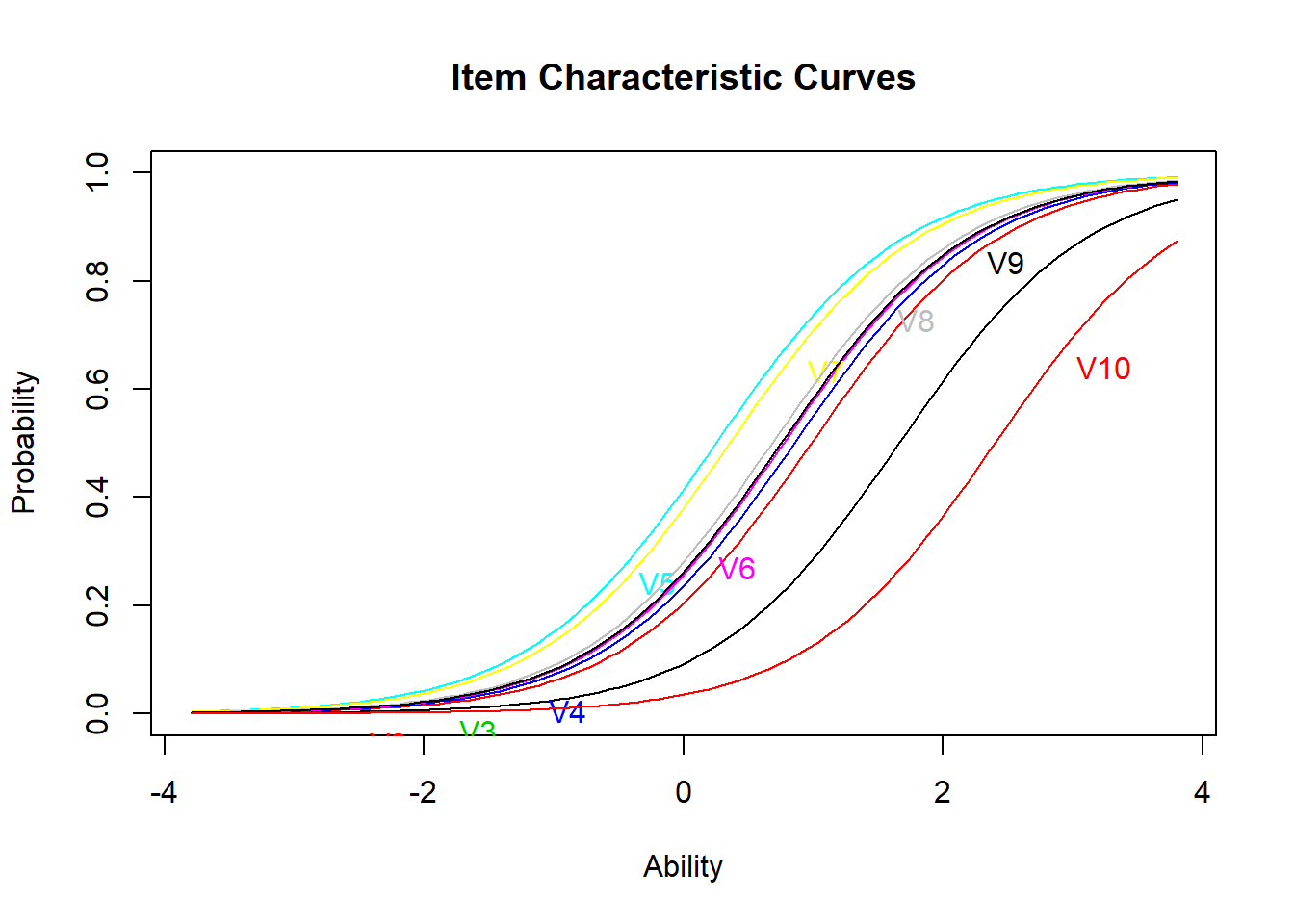From this plot, we see that item 10 is the most difficult item (it’s curve is farthest to the right), and item 5 is the easiest (it’s curve is farthest to the left). The same conclusions can be drawn by checking the difficulty estimates above.

## 2.3 Step 3: Plot the item information curves for all 10 items, then the whole test

Item information curves show how much “information” about the latent trait ability an item gives. Mathematically, these are the 1st derivatives of the ICCs. Item information curves peak at the difficulty value (point where the item has the highest discrimination), with less information at ability levels farther from the difficulty estimate.

Practially speaking, we can see how a very difficult item will provide very little information about persons with low ability (because the item is already too hard), and very easy items will provide little information about persons with high ability levels.

plot(PL1.rasch,type=c("IIC"))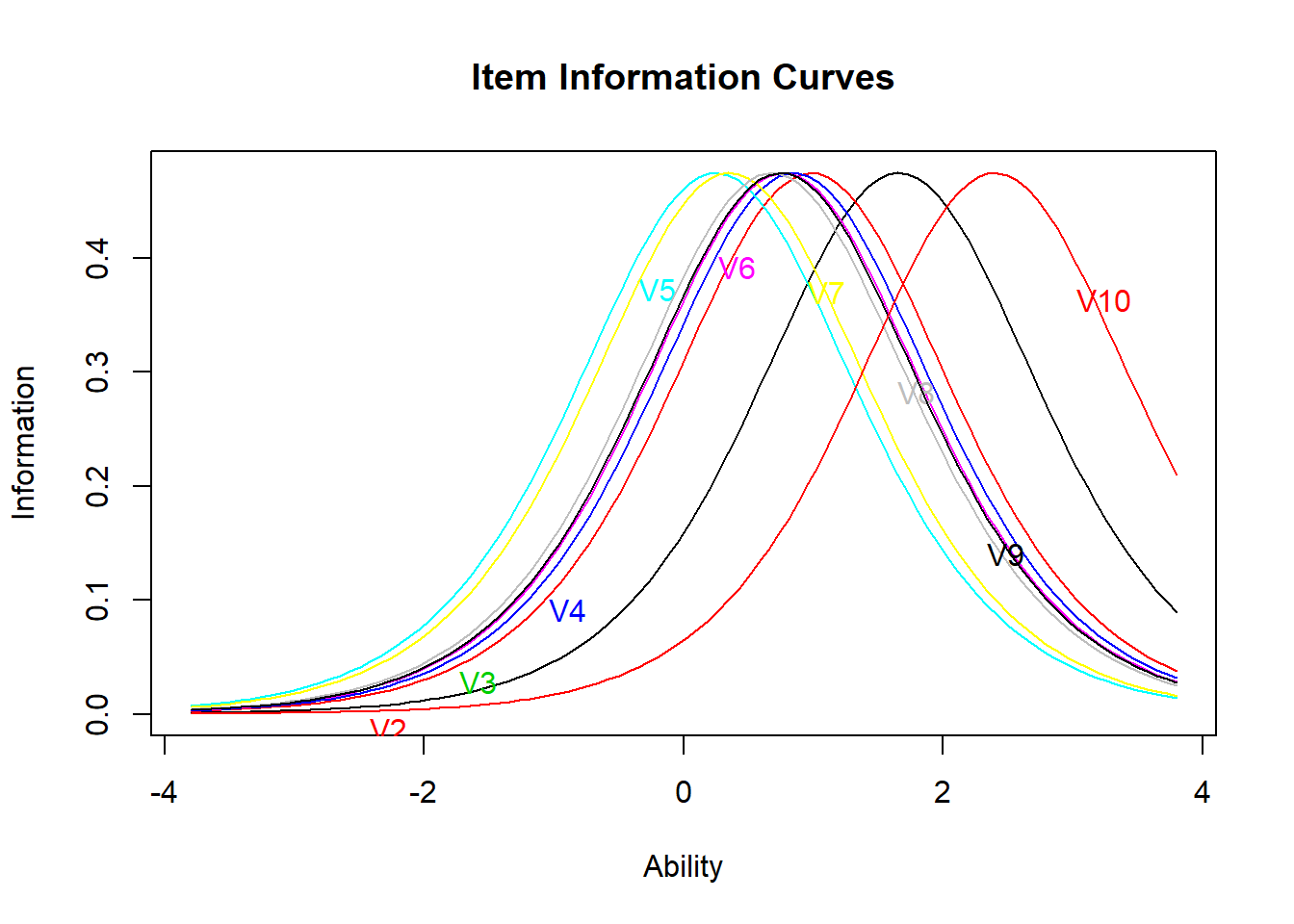Similar to the ICCs, we see that item 10 provides the most information about high ability levels (the peak of its IIC is farthest to the right) and item 5 provides the most information about lower ability levels (the peak of its IIC is farthest to the left). We have seen that all ICCs and IICs for the items have the same shape in the 1PL model (i.e., all items are equally good at providing information about the latent trait). In the 2PL and 3PL models, we will see that this does not have to be the case.

Next, we plot the information curve for the whole test. This is simply the sum of the individual IICs above. Ideally, we want a test which provides fairly good covereage of a wide range of latent ability levels. Otherwise, the test is only good at identifying a limited range of ability levels.

We see that this test provides the most information about slightly-higher-than-average ability levels (the peak is around ability level $$\theta=.5$$), and less information about very high and very low ability levels.

plot(PL1.rasch,type=c("IIC"),items=c(0))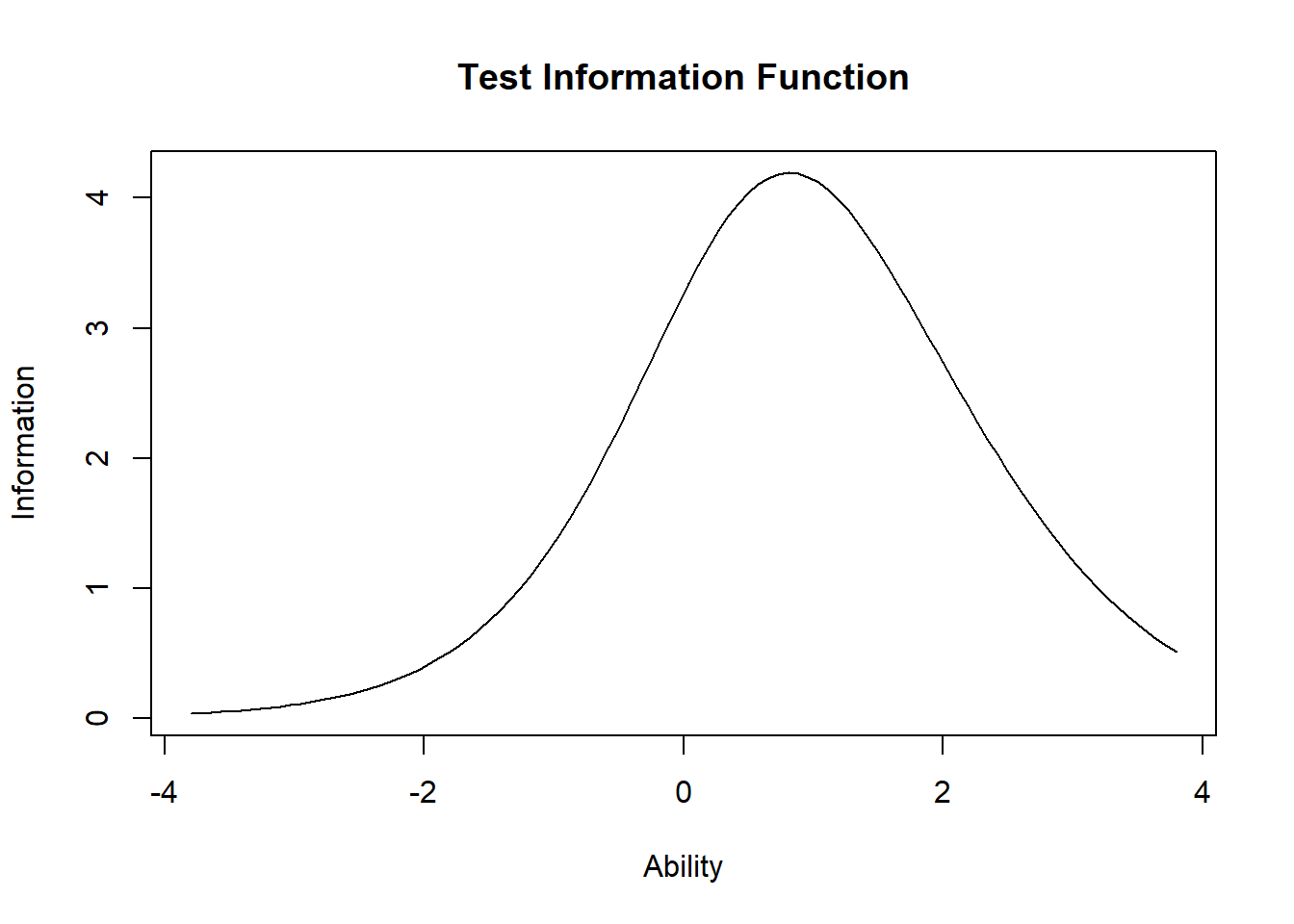## 2.4 Step 4: Test the fit of the 1PL model

We run the item.fit function to test whether individual items fit the 1PL model.

item.fit(PL1.rasch,simulate.p.value=T)
##
## Item-Fit Statistics and P-values
##
## Call:
## rasch(data = irtdat)
##
## Alternative: Items do not fit the model
## Ability Categories: 10
## Monte Carlo samples: 100
##
##         X^2 Pr(>X^2)
## V1  12.1830   0.5941
## V2  15.1135    0.505
## V3  11.9995   0.7327
## V4  21.0226   0.1782
## V5  15.3784   0.5545
## V6  14.7103   0.5842
## V7   4.0974        1
## V8  23.3690   0.1683
## V9  26.4597   0.0495
## V10 25.7195   0.0693

We see from this that items 8, 9, and 10 perhaps do not fit the 1PL model so well (small p-values). This is an indication that we should consider a different IRT model.

## 2.5 Step 5: Estimate ability scores & plot

Next, we can take the results of our 1PL model and estimate the latent ability scores of the participants.

We estimate the ability scores with the factor.scores() function in the ltm package.

theta.rasch<-ltm::factor.scores(PL1.rasch)
summary(theta.rasch$score.dat$z1)
##    Min. 1st Qu.  Median    Mean 3rd Qu.    Max.
## -1.0376  0.1770  0.4631  0.5542  0.9988  2.2795

We can also plot the density curve of the estimated ability scores:

plot(theta.rasch)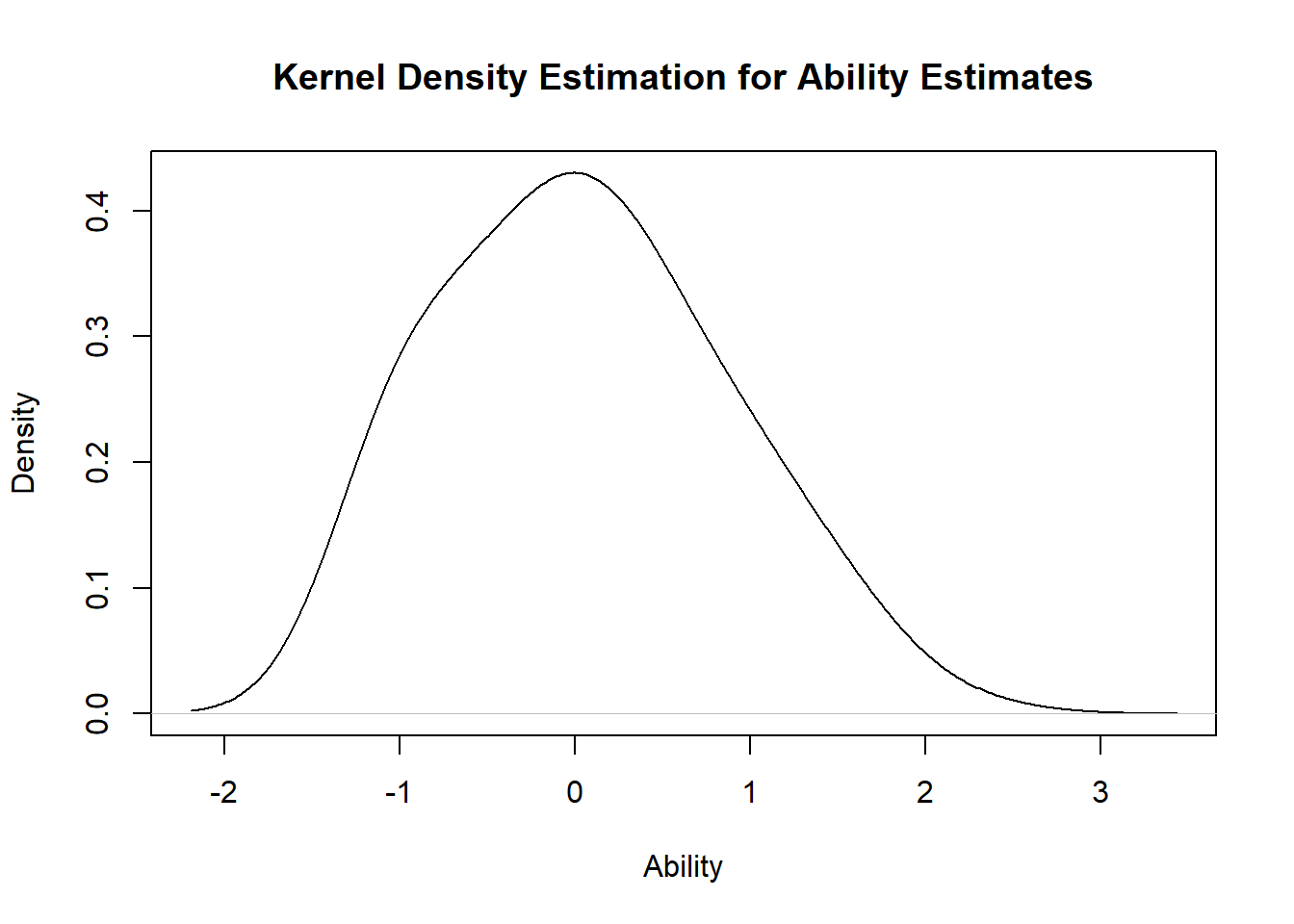We see that the mean of ability scores is around 0, and the standard deviation about 1 (these are by definition-estimated ability scores are standardized). The curve is approximately normal-shaped.

## 2.6 Step 6: Test for unidimensionality

unidimTest(PL1.rasch,irtdat)
##
## Unidimensionality Check using Modified Parallel Analysis
##
## Call:
## rasch(data = irtdat)
##
## Matrix of tertachoric correlations
##         V1     V2     V3     V4     V5     V6     V7     V8     V9    V10
## V1  1.0000 0.3476 0.3357 0.3959 0.4449 0.4422 0.5215 0.3278 0.4158 0.3403
## V2  0.3476 1.0000 0.3124 0.3945 0.3909 0.3664 0.2408 0.3352 0.4293 0.4101
## V3  0.3357 0.3124 1.0000 0.3933 0.2970 0.3734 0.3011 0.4434 0.4040 0.4058
## V4  0.3959 0.3945 0.3933 1.0000 0.3853 0.5101 0.3035 0.4368 0.5135 0.6024
## V5  0.4449 0.3909 0.2970 0.3853 1.0000 0.3370 0.3526 0.4138 0.5149 0.3979
## V6  0.4422 0.3664 0.3734 0.5101 0.3370 1.0000 0.3137 0.4146 0.4801 0.4795
## V7  0.5215 0.2408 0.3011 0.3035 0.3526 0.3137 1.0000 0.3311 0.2054 0.1714
## V8  0.3278 0.3352 0.4434 0.4368 0.4138 0.4146 0.3311 1.0000 0.5069 0.4533
## V9  0.4158 0.4293 0.4040 0.5135 0.5149 0.4801 0.2054 0.5069 1.0000 0.6099
## V10 0.3403 0.4101 0.4058 0.6024 0.3979 0.4795 0.1714 0.4533 0.6099 1.0000
##
## Alternative hypothesis: the second eigenvalue of the observed data is substantially larger
##          than the second eigenvalue of data under the assumed IRT model
##
## Second eigenvalue in the observed data: 0.6863
## Average of second eigenvalues in Monte Carlo samples: 0.4899
## Monte Carlo samples: 100
## p-value: 0.0297

The test is borderline significant at alpha=0.01 (p=0.0198), so unidimensionality (the idea that we’re measuring a single trait $$\theta$$ here) is rejected. Since the 1PL model did not fit very well, we should consider fitting the data to alternative IRT models. The poor fit is perhaps not surprising, given that we know the data were simulated using a 2PL model, and we are not accounting for item differences in discriminability.

# 3 2-parameter logistic (2PL) IRT model

The item response function for the 2-parameter logistic IRT model is: $P(X=1|\theta,a,b)=\frac{e^{a(\theta-b)}}{1+e^{a(\theta-b)}}$ The IRF describes the probability that an individual with latent ability level $$\theta$$ endorses an item ($$X=1$$) with two item characteristc paramters:
1. $$b$$ is the item difficulty (same as 1PL model). Item difficulty is reflected in the position of the item characteristic curve along the x-axis (latent trait ablity).
2. $$a$$ is the item discriminability. Discriminability is how well an item is able to discriminate between persons with different ability levels. Item discriminability is reflected in the steepness of the slope of the item characteristic curves.

## 3.1 Step 1: Fit the 2PL model

We fit the 2PL model with ltm.

PL2.rasch<-ltm(irtdat~z1)
summary(PL2.rasch)
##
## Call:
## ltm(formula = irtdat ~ z1)
##
## Model Summary:
##   log.Lik      AIC      BIC
##  -2575.88 5191.759 5276.052
##
## Coefficients:
##             value std.err z.vals
## Dffclt.V1  1.6318  0.1920 8.4979
## Dffclt.V2  1.1022  0.1550 7.1098
## Dffclt.V3  0.8210  0.1270 6.4627
## Dffclt.V4  0.7778  0.1015 7.6660
## Dffclt.V5  0.2550  0.0925 2.7571
## Dffclt.V6  0.7372  0.1046 7.0473
## Dffclt.V7  0.4766  0.1339 3.5593
## Dffclt.V8  0.6493  0.0994 6.5356
## Dffclt.V9  0.6485  0.0889 7.2943
## Dffclt.V10 1.9257  0.2027 9.5001
## Dscrmn.V1  1.4183  0.2315 6.1265
## Dscrmn.V2  1.1445  0.1741 6.5733
## Dscrmn.V3  1.1910  0.1736 6.8621
## Dscrmn.V4  1.6444  0.2279 7.2157
## Dscrmn.V5  1.3398  0.1851 7.2367
## Dscrmn.V6  1.4973  0.2070 7.2335
## Dscrmn.V7  0.8748  0.1430 6.1185
## Dscrmn.V8  1.5133  0.2070 7.3116
## Dscrmn.V9  1.8716  0.2587 7.2357
## Dscrmn.V10 2.1060  0.4226 4.9834
##
## Integration:
## method: Gauss-Hermite
##
## Optimization:
## Convergence: 0
## quasi-Newton: BFGS

All of the item characteristic estimates (difficultly and discrimination) have significant z-values (i.e. greater than 1.65, against the null hypothesis that parameters = 0). For example, for Item 1, the estimated difficulty is $$b=1.63 (z=8.50)$$ and the estimated discriminability is $$a=1.42 (z=6.13)$$. Higher difficulty values indicate that the item is harder (i.e., higher latent ability to answer correctly); higher discriminability estimates indicate that the item has better ability to tell the difference between different levels of latent ability. These will both be made clearer in the ICC plots.

## 3.2 Step 2: Plot the item characteristic curves of all 10 items

plot(PL2.rasch,type=c("ICC"))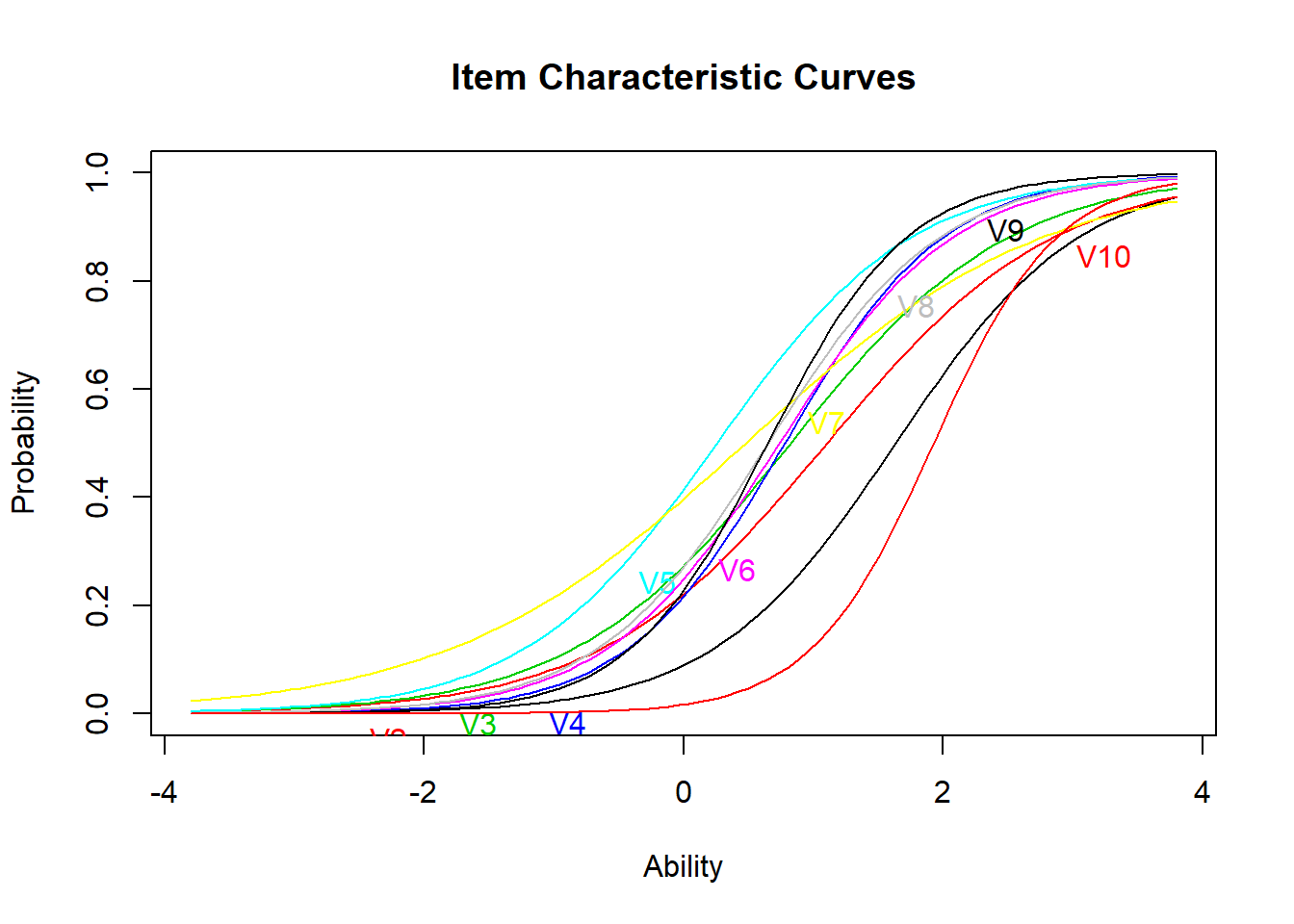Unlike the ICCs for the 1PL model, the ICCs for the 2PL model do not all have the same shape. Item curves which are more “spread out” indicate lower discriminability (i.e., that individuals of a range of ability levels have some probability of getting the item correct). Compare this to an item with high discriminability (steep slope): for this item, we have a better estimate of the individual’s latent ability based on whether they got the question right or wrong.

A note about difficulty: Because of the differing slopes, the rank-order of item difficulty changes across different latent ability levels. We can see that item 10 is generally still the most difficult item (i.e. lowest probability of getting correct for most latent trait values, up until about $$\theta=2$$). Items 5 and 6 are roughly the easiest, switching in the rank order somewhere around $$\theta=-.25$$.

## 3.3 Step 3: Plot the item information curves for all 10 items, then the whole test

plot(PL2.rasch,type=c("IIC"))